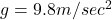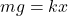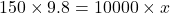## A 150kg person stands on a compression spring with spring constant 10, 000 N/m and norminal.Length of 0.50.What is the togal length of the l

Question

A 150kg person stands on a compression spring with spring constant 10, 000 N/m and norminal.Length of 0.50.What is the togal length of the loaded spring?

in progress 0
2 months 2021-07-29T21:07:00+00:00 1 Answers 3 views 0

Total length of spring 0.647 m

Explanation:

We have given mass of the person m = 150 kg

Acceleration due to gravitySpring constant k = 10000 N/m

Nominal length of spring = 0.50

According to hook’s lawx = 0.147 m

So total length of spring = 0.50+0.147 = 0.647 m